# Degenerate hyperbolic equation

(diff) ← Older revision | Latest revision (diff) | Newer revision → (diff)

A partial differential equation(*)

where the function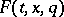satisfies the following condition: The roots of the polynomial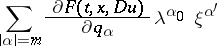are real for all real, and there exist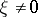,,, and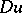for which some of the roots either coincide or the coefficient of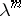vanishes. Hereis an independent variable which is often interpreted as time;is an-dimensional vector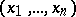;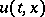is the unknown function;and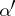are multi-indices,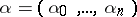,;is a vector with components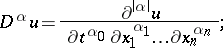only derivatives of an order not exceedingenter in equation (*); the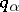are the components of a vector;is an-dimensional vector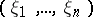; and.